# Methods with Parameters

Frequently in order to solve a problem, the method by which we do this needs additional information, which depends on its purpose. This is precisely the information that the method parameters are, and its behavior depends on them.

## Using Parameters in Methods

As we observed above, the parameters can be zero, one or more. When declaring them you should divide them with a comma. They can be of any type (int, string etc.), and there is an example below to show how they can be used by the method.

We declare the method and its list of parameters, then we write the code that the method executes.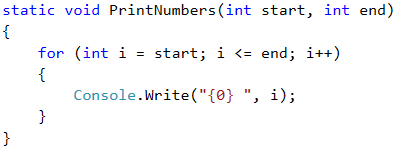After that call the method and give it particular values: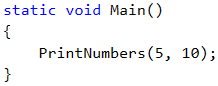When declaring parameters, we can use various types of variables, and we should be careful that every parameter has a type and name. It is important to note that when calling the method, we must pass to it values for the parameters in the order, in which they are declared. If the parameters are first int and after that string, when calling the method, we can't give it first a string and then int. We can only change places of given parameters if we write the name of the parameter beforehand, as you will see below in one of the examples. This is generally not a good practice!

Let's look at the example for declaring a method, which has several parameters of different types.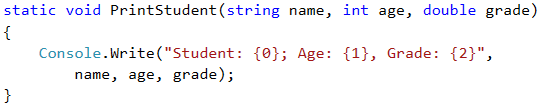## Example: Sign of an Integer

Create a method that prints the sign of an integer n.

### Sample Input and Output

Input Output
2 The number 2 is positive.
-5 The number -5 is negative.
0 The number 0 is zero.

### Hints and Guidelines

The first step is creating a method and giving it a descriptive name, e.g. PrintSign. This method will have only one parameter of int type.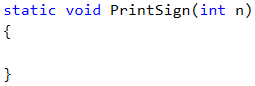The next step is implementing the logic by which the program will check what the sign of the number is. You can see from the examples that there are three cases – the number is larger than, equal to or lower than zero, which means that we'll make three verifications in our method.

The next step is to read the input number and to call the new method from the body of the Main method.## Optional Parameters

C# supports using optional parameters. They allow missing parameters when calling the method. Their declaring is done by providing default value in the description of the parameter.

The following example illustrates using optional parameters: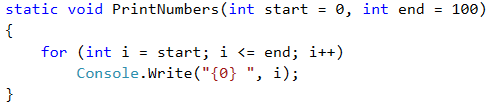The shown method PrintNumbers can be called in one of several ways: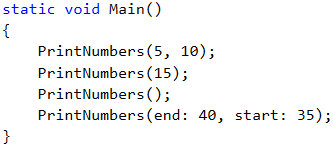## Example: Printing a Triangle

Create a method which prints a triangle as in the examples.

### Sample Input and Output

Input Output Input Output
3 1
1 2
1 2 3
1 2
1
4 1
1 2
1 2 3
1 2 3 4
1 2 3
1 2
1

### Hints and Guidelines

Before creating a method to print a row with a given beginning and an end, we must read the input number from the console. After that we choose a meaningful name, which describes its purpose, e.g. PrintLine, and implement it.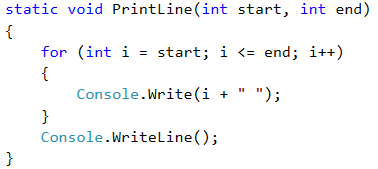From the drawing exercises we remember that it is good practice to divide the figure into several parts. To make it easier we will divide the triangle into three parts – upper, middle and lower.

The next step is to print the upper half of the triangle using a loop:Then we print the middle part: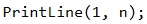In the end we print the lower part of the triangle, but this time the loop step decreases.## Example: Draw a Filled Square

Print on the console a filled square with side n, as in the examples below.

### Sample Input and Output

Input Output Input Output
4 --------
-\/\/\/-
-\/\/\/-
--------
5 ----------
-\/\/\/\/-
-\/\/\/\/-
-\/\/\/\/-
----------

### Hints and Guidelines

The first step is to read the input from the console. After that we need to create a method, which will print the first and the last rows because they are the same. Let's remember that we must give it a descriptive name and give it as a parameter the length of the side. We will use the constructor new string.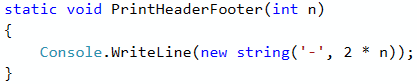Our next step is to create a method that will draw the middle rows on the console. Again, give it a descriptive name i.e. PrintMiddleRow.Finally, call the methods in the Main() method of the program in order to draw the whole square: